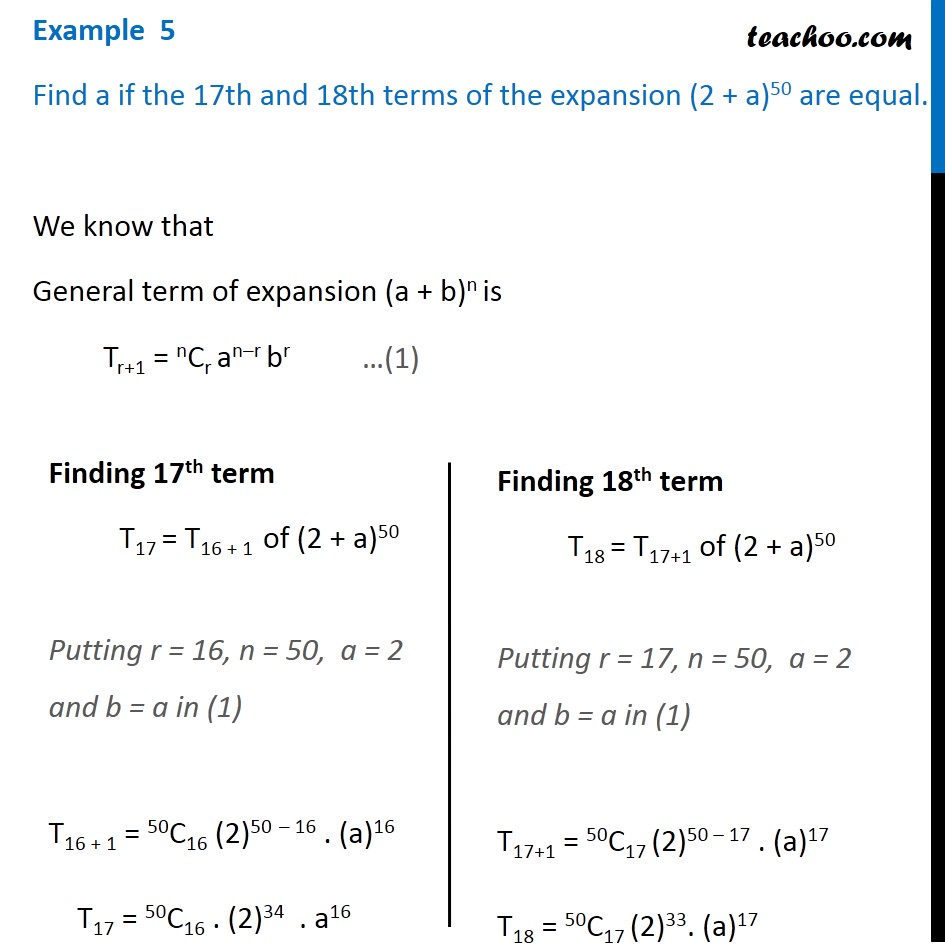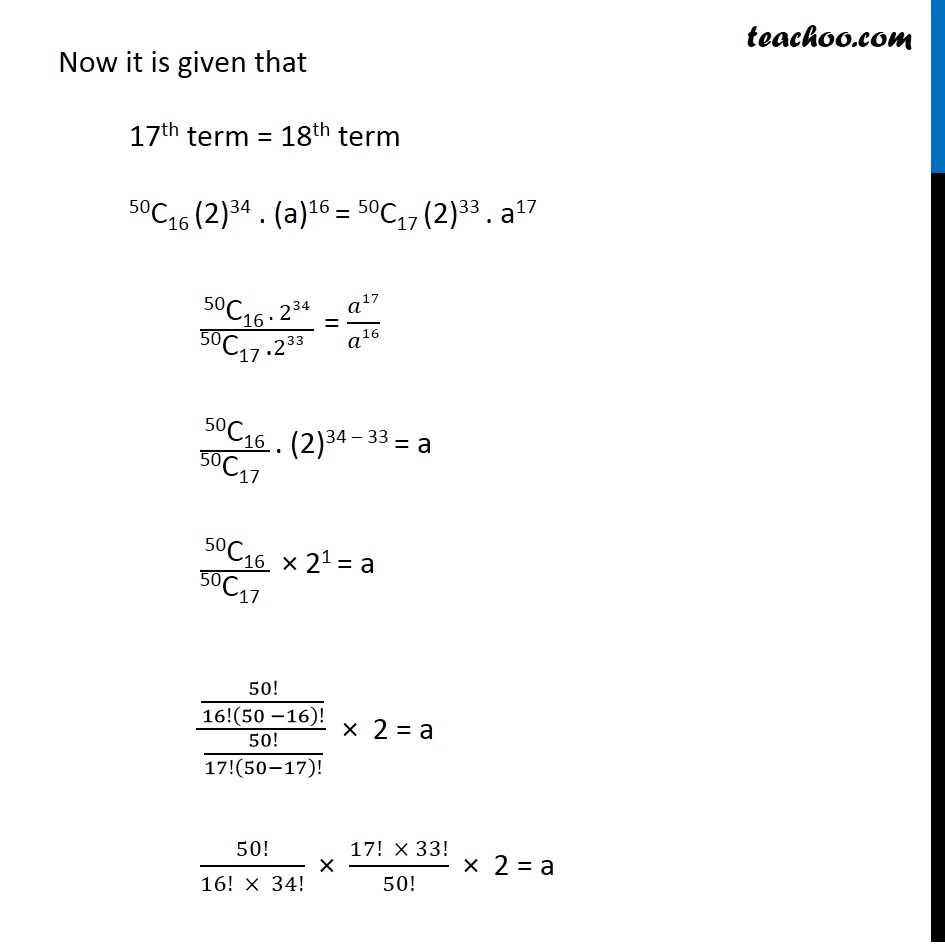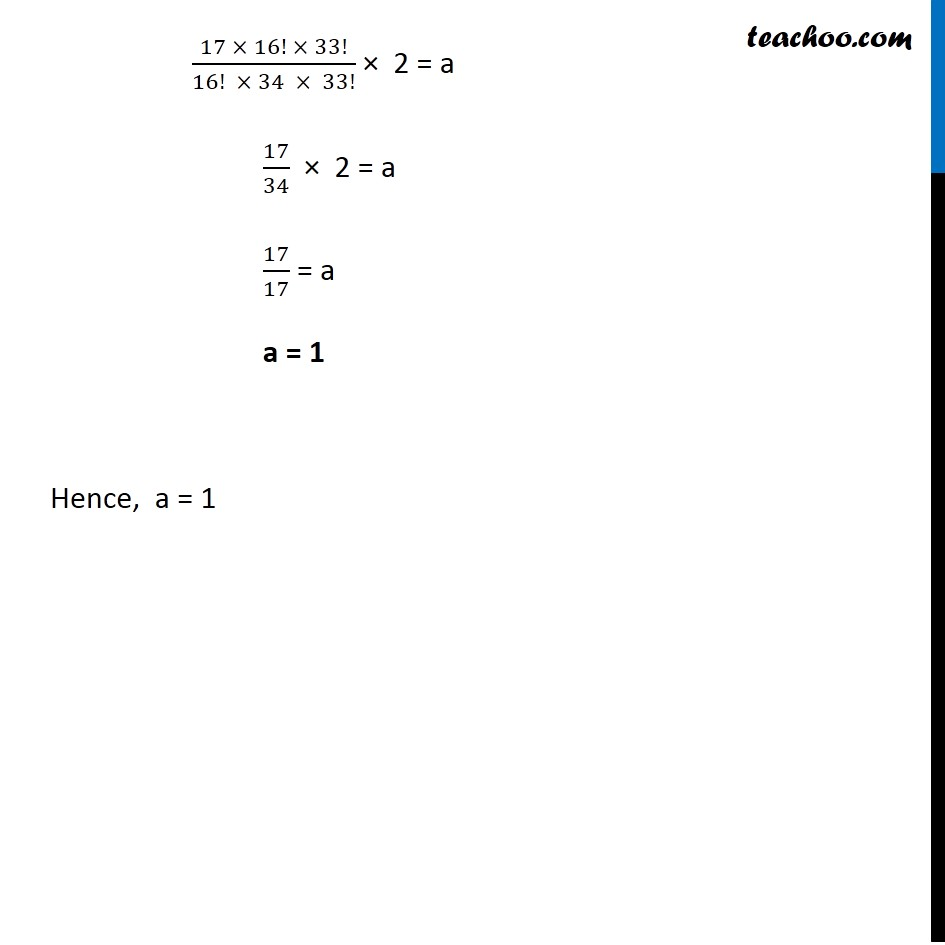Examples

Chapter 7 Class 11 Binomial Theorem
Serial order wiseLearn in your speed, with individual attention - Teachoo Maths 1-on-1 Class

### Transcript

Question 1 Find a if the 17th and 18th terms of the expansion (2 + a)50 are equal. We know that General term of expansion (a + b)n is Tr+1 = nCr an–r br Finding 17th term T17 = T16 + 1 of (2 + a)50 Putting r = 16, n = 50, a = 2 and b = a in (1) T16 + 1 = 50C16 (2)50 – 16 . (a)16 T17 = 50C16 . (2)34 . a16 Finding 18th term T18 = T17+1 of (2 + a)50 Putting r = 17, n = 50, a = 2 and b = a in (1) T17+1 = 50C17 (2)50 – 17 . (a)17 T18 = 50C17 (2)33. (a)17 Now it is given that 17th term = 18th term 50C16 (2)34 . (a)16 = 50C17 (2)33 . a17 ("50C16 " . 234)/("50C17 ." 233" " ) = 𝑎17/𝑎16 "50C16" /"50C17 " . (2)34 – 33 = a "50C16" /"50C17 " × 21 = a (50!/16!(50 −16)!)/(50!/17!(50−17)!) × 2 = a 50!/(16! × 34!) × (17! × 33!)/50! × 2 = a (17 × 16! × 33!)/(16! × 34 × 33!) × 2 = a 17/34 × 2 = a 17/17 = a a = 1 Hence, a = 1